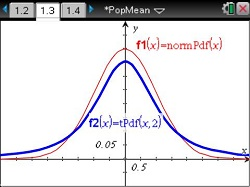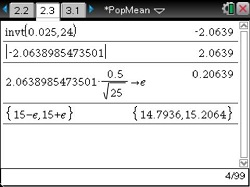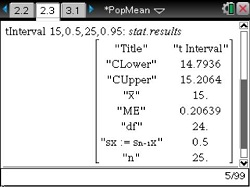# Activities

••• ##### Subject Area

• Math: Statistics: Statistical Inference

• ##### Author9-12

15 Minutes

• ##### Device
• TI-Nspire™ Navigator™
• TI-Nspire™
• TI-Nspire™ CAS
• ##### Software

TI-Nspire™
TI-Nspire™ CAS

3.0

• ##### Report an Issue

Population Mean: σ unknown

#### Activity Overview

Students calculate confidence intervals to estimate the true population mean when the standard deviation of the population is not known.

#### Key Steps

•Students will be introduced to the assumptions needed to use the t distribution. They will investigate, by graphing, the relationship between a normal distribution and the t distribution.

•Students are presented with a problem to calculate a confidence interval. They will use a Calculator page to compute the interval, finding the t-value and the error.

•As an extension, students are given a data set and asked to construct three confidence intervals. They will be able to use either method from the activity.